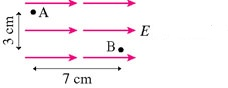# Problem: a. Which point, A or B, has a larger electric potentialb. What is the potential difference between A and B? Assume that E=1500V/m

###### FREE Expert Solution

a.

Point A is at a larger electric potential.

90% (380 ratings)###### Problem Detailsa. Which point, A or B, has a larger electric potential

b. What is the potential difference between A and B? Assume that E=1500V/m